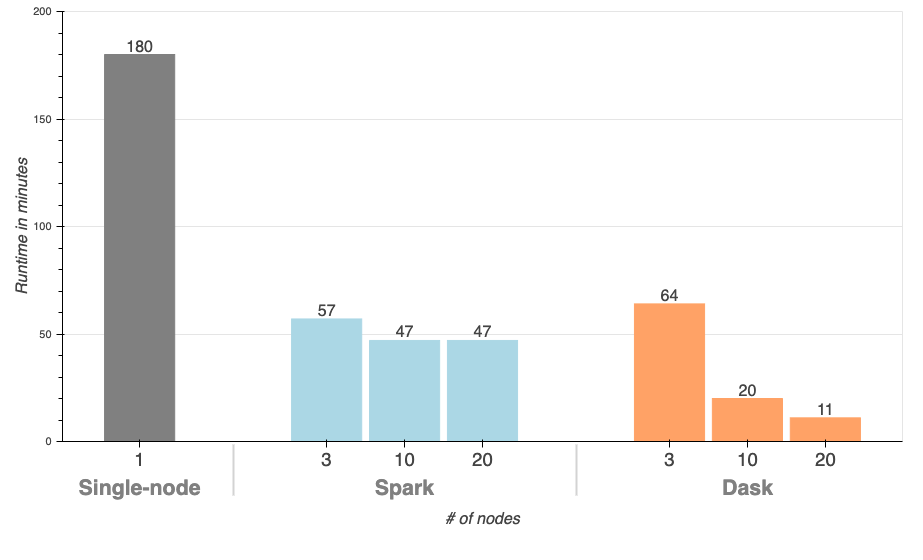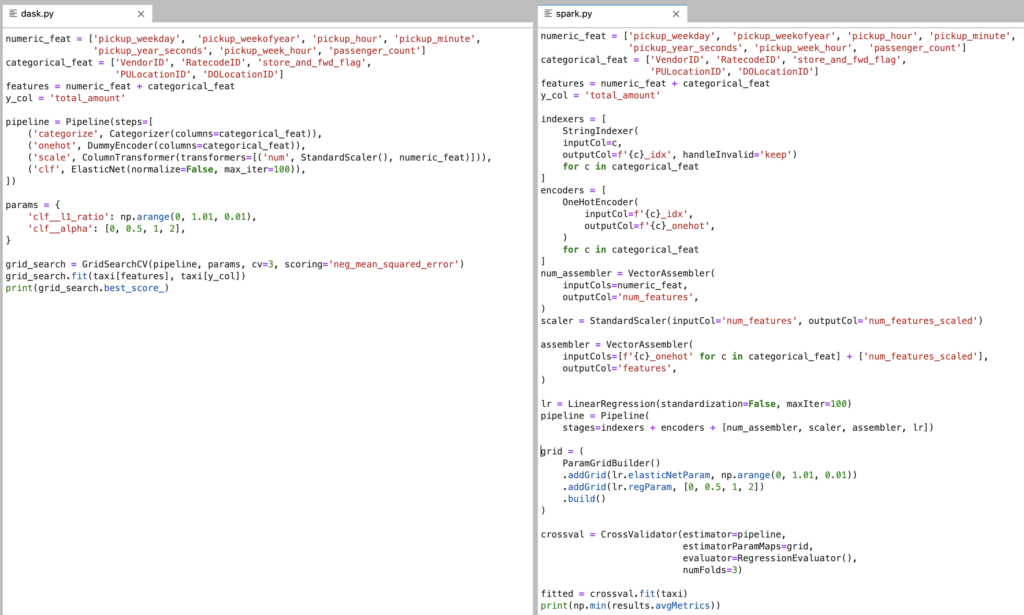# Supercharging Hyperparameter Tuning with Dask

The distributed computing framework Dask is great for hyperparameter tuning, since you can train different parameter sets concurrently.TL;DR: Dask improves scikit-learn parameter search speed by over 16x, and Spark by over 4x.Hyperparameter tuning is a crucial, and often painful, part of building machine learning models. Squeezing out each bit of performance from your model may mean the difference of millions of dollars in ad revenue or life-and-death for patients in healthcare models. Even if your model takes one minute to train, you can end up waiting hours for a grid search to complete (think a 10×10 grid, cross-validation, etc.). Each time you wait for a search to finish breaks an iteration cycle and increases the time it takes to produce value with your model. Shortly put:

• Faster runtime means more iterations to improve accuracy before your deadline
• Faster runtime means quicker delivery so you can tackle another project
• Both bullet points mean driving value to the bottom line of your organization

In this post, we’ll see show how you can improve the speed of your hyperparameter search by over 16x by replacing a few lines of your scikit-learn pipeline with Dask code on Saturn Cloud. This turns a traditionally overnight parameter search to a matter of waiting a few seconds. We also try a comparable grid search with Apache Spark which requires significantly more code change while still being much slower than Dask.

Dask is a flexible and robust parallel computing framework built-in, and for, Python. It works with common data structures such as Arrays and DataFrames, but can also be used to parallelize complex operations that do not fit nicely into those. In fact, the parallel Arrays and DataFrames are actually collections of familiar `numpy` and `pandas` objects, and have matching APIs. This way, data scientists do not need to learn entirely new frameworks to be able to execute their code on big data. (To learn more about Dask, read here.)

## Experiment setup

We will use the publicly available NYC Taxi dataset and train a linear regression model that can predict the fare amount of a taxi ride using attributes related to rider pickup.

First, we’ll walk through the data loading and grid search using single-node Python packages (`pandas` and `scikit-learn`), and then highlight what changes are required to parallelize the grid search with Dask or Spark. All three workloads perform the same grid search with the same data, and we will refer to them throughout the article as Single-node (for single-node Python), Dask (for Dask cluster), and Spark (For Spark cluster).

### Hardware

For all tasks, we use r5.2xlarge instances from AWS (8 cores, 64GB RAM). For Python, we only use one node, and for Spark and Dask, we run the workload on clusters with different numbers of worker nodes to track the runtime (3, 10, and 20).

Spark clusters are managed using Amazon EMR, while Dask clusters are managed using Saturn Cloud.

### Just show me the results!

If you want to skip the code and see the performance improvements, jump down to the Results section.

## Single-node workflow

First, load the data! We randomly sample the data for benchmark purposes.

``````import pandas as pd
import numpy as np
import datetime

's3://nyc-tlc/trip data/yellow_tripdata_2019-01.csv',
parse_dates=['tpep_pickup_datetime', 'tpep_dropoff_datetime'],
).sample(frac=0.1, replace=False)
``````

Then, create some features:

``````taxi['pickup_weekday'] = taxi.tpep_pickup_datetime.dt.weekday
taxi['pickup_weekofyear'] = taxi.tpep_pickup_datetime.dt.weekofyear
taxi['pickup_hour'] = taxi.tpep_pickup_datetime.dt.hour
taxi['pickup_minute'] = taxi.tpep_pickup_datetime.dt.minute
taxi['pickup_year_seconds'] = (taxi.tpep_pickup_datetime - datetime.datetime(2019, 1, 1, 0, 0, 0)).dt.seconds
taxi['pickup_week_hour'] = (taxi.pickup_weekday * 24) + taxi.pickup_hour
taxi['passenger_count'] = taxi.passenger_count.astype(float).fillna(-1)
taxi = taxi.fillna(value={'VendorID': 'missing', 'RatecodeID': 'missing', 'store_and_fwd_flag': 'missing' })

# keep track of column names for pipeline steps
numeric_feat = ['pickup_weekday',  'pickup_weekofyear', 'pickup_hour', 'pickup_minute', 'pickup_year_seconds', 'pickup_week_hour',  'passenger_count']
categorical_feat = ['VendorID', 'RatecodeID', 'store_and_fwd_flag', 'PULocationID', 'DOLocationID']
features = numeric_feat + categorical_feat
y_col = 'total_amount'
``````

We are using a scikit-learn Elastic Net model, which can perform L1, L2 and ElasticNet regularization based on the `l1_ratio` parameter. We’ll also try a few values of `alpha`, creating a grid with 404 items and 3-fold cross-validation, resulting in 1,212 model fits for the search.

As we’re training a linear model, we need to one-hot encode the categorical features and scale the numeric features. Note the `n_jobs=-1` parameter when defining the `GridSearchCV` instructs scikit-learn to parallelize the model training across all the cores in the machine (this doesn’t use Dask yet, as the single-node parallelization comes out-of-the-box with scikit-learn).

``````from sklearn.pipeline import Pipeline
from sklearn.linear_model import ElasticNet
from sklearn.compose import ColumnTransformer
from sklearn.preprocessing import StandardScaler, OneHotEncoder
from sklearn.model_selection import GridSearchCV

pipeline = Pipeline(steps=[
('preprocess', ColumnTransformer(transformers=[
('num', StandardScaler(), numeric_feat),
('cat', OneHotEncoder(handle_unknown='ignore', sparse=False), categorical_feat),
])),
('clf', ElasticNet(normalize=False, max_iter=100)),
])

# this is our grid
params = {
'clf__l1_ratio': np.arange(0, 1.01, 0.01),
'clf__alpha': [0, 0.5, 1, 2],
}

grid_search = GridSearchCV(pipeline, params, cv=3, n_jobs=-1, scoring='neg_mean_squared_error')
``````

Finally, we can run the grid search and retrieve the best score:

``````grid_search.fit(taxi[features], taxi[y_col])
print(grid_search.best_score_)
``````

If you were to run this on a similarly-sized machine as we did it would take approximately 3 hours.

Dask will run great on a single-node and can scale to clusters with thousands of nodes. To start using Dask, we need to initialize a client, and in this case we will set up our cluster using Saturn Cloud’s `SaturnCluster`.

``````from dask.distributed import Client

cluster = SaturnCluster(n_workers=20)
client = Client(cluster)
``````

The only change we need to make when reading the data is to use the `dask.dataframe` package instead of `pandas`All the feature engineering code remains exactly the same because Dask Dataframes implement the pandas API.

``````import dask.dataframe as dd

's3://nyc-tlc/trip data/yellow_tripdata_2019-01.csv',
parse_dates=['tpep_pickup_datetime', 'tpep_dropoff_datetime'],
storage_options={'anon': True},
).sample(frac=0.1, replace=False)
``````

Then to create our pipeline and grid search:

``````from dask_ml.compose import ColumnTransformer
from dask_ml.preprocessing import StandardScaler, DummyEncoder, Categorizer

# Dask has slightly different way of one-hot encoding
pipeline = Pipeline(steps=[
('categorize', Categorizer(columns=categorical_feat)),
('onehot', DummyEncoder(columns=categorical_feat)),
('scale', ColumnTransformer(transformers=[('num', StandardScaler(), numeric_feat)],
remainder='passthrough')),
('clf', ElasticNet(normalize=False, max_iter=100)),
])

# params are same as used in single node workflow
params = {
'clf__l1_ratio': np.arange(0, 1.01, 0.01),
'clf__alpha': [0, 0.5, 1, 2],
}

grid_search = GridSearchCV(pipeline, params, cv=3, scoring='neg_mean_squared_error')
``````

Note that Dask has a couple different classes for preprocessing and `GridSearchCV`, which are used to speed up pre-processing and avoid unnecessary re-computation during the grid search. The pipeline and estimator (`ElasticNet`) classes are used directly from scikit-learn.

We can fit the grid search the same way as we did with the single-node scikit-learn:

``````grid_search.fit(taxi[features], taxi[y_col])
print(grid_search.best_score_)
``````

Running this grid search using 20 nodes results in a runtime of 11 minutes! That a 16x improvement by just changing ~10 lines of code. Let me be dramatic and say that again.

16x improvement by changing 10 lines of code!

## Spark

Apache Spark is a big data processing engine built in Scala with a Python interface that calls down to the Scala/JVM code. It’s a staple in the Hadoop processing ecosystem, built around the MapReduce paradigm, and has interfaces for DataFrames as well as machine learning.

To run our workload with Spark, we need to refactor our Python code to use Spark’s DataFrame as well as Spark ML pipelines, preprocessing, and model classes.

``````from pyspark.sql import SparkSession

spark = SparkSession.builder.getOrCreate()

inferSchema=True,
timestampFormat='yyyy-MM-dd HH:mm:ss',
).sample(fraction=0.1, withReplacement=False)
``````

The feature engineering looks slightly different than pandas:

``````import pyspark.sql.functions as F
import pyspark.sql.types as T

taxi = taxi.withColumn('pickup_weekday', F.dayofweek(taxi.tpep_pickup_datetime).cast(T.DoubleType()))
taxi = taxi.withColumn('pickup_weekofyear', F.weekofyear(taxi.tpep_pickup_datetime).cast(T.DoubleType()))
taxi = taxi.withColumn('pickup_hour', F.hour(taxi.tpep_pickup_datetime).cast(T.DoubleType()))
taxi = taxi.withColumn('pickup_minute', F.minute(taxi.tpep_pickup_datetime).cast(T.DoubleType()))
taxi = taxi.withColumn('pickup_year_seconds',
(F.unix_timestamp(taxi.tpep_pickup_datetime) -
F.unix_timestamp(F.lit(datetime.datetime(2019, 1, 1, 0, 0, 0)))).cast(T.DoubleType()))
taxi = taxi.withColumn('pickup_week_hour', ((taxi.pickup_weekday * 24) + taxi.pickup_hour).cast(T.DoubleType()))
taxi = taxi.withColumn('passenger_count', F.coalesce(taxi.passenger_count, F.lit(-1)).cast(T.DoubleType()))
taxi = taxi.fillna()
# Spark ML expects a "label" column for the dependent variable
taxi = taxi.withColumn('label', taxi.total_amount)
``````

Then, we set up our pre-processing pipeline and grid search. Spark ML expects all features in a single vector column, so we use `VectorAssembler` to collect all our processed columns.

``````from pyspark.ml.regression import LinearRegression
from pyspark.ml.tuning import CrossValidator, ParamGridBuilder
from pyspark.ml.evaluation import RegressionEvaluator
from pyspark.ml.feature import OneHotEncoder, StringIndexer, VectorAssembler, StandardScaler
from pyspark.ml.pipeline import Pipeline

indexers = [
StringIndexer(
inputCol=c,
outputCol=f'_idx', handleInvalid='keep')
for c in categorical_feat
]
encoders = [
OneHotEncoder(
inputCol=f'_idx',
outputCol=f'_onehot',
)
for c in categorical_feat
]
num_assembler = VectorAssembler(
inputCols=numeric_feat,
outputCol='num_features',
)
scaler = StandardScaler(inputCol='num_features', outputCol='num_features_scaled')

assembler = VectorAssembler(
inputCols=[f'_onehot' for c in categorical_feat] + ['num_features_scaled'],
outputCol='features',
)

lr = LinearRegression(standardization=False, maxIter=100)
pipeline = Pipeline(
stages=indexers + encoders + [num_assembler, scaler, assembler, lr])

# this is our grid
grid = (
ParamGridBuilder()
.build()
)

crossval = CrossValidator(estimator=pipeline,
estimatorParamMaps=grid,
evaluator=RegressionEvaluator(),
numFolds=3)
``````

``````fitted = crossval.fit(taxi)
print(np.min(results.avgMetrics))  # min because metric is RMSE
``````

On a 20 node cluster, this takes approximately 47 minutes.

Here is a side by side look at the grid search code to give you a sense of how much easier Dask is in this example.

Code Volume: Dask (left) and Spark (right)## Results

We ran a hyperparameter search with 404 configurations and 3 folds of cross-validation, using an Elastic Net model to predict taxi trip duration from the NYC taxi dataset. We started off with a single-node Python implementation and transferred that to a cluster using Dask and Spark. We can see from the table below that the Dask search was drastically faster than the single-node and Spark cluster versions, while requiring minimal code changes.

SystemRuntimeLOC changed
Single-node3 hours
Spark47 minutes100

We ran the Dask and Spark workloads on clusters of various sizes to see how a larger cluster improves parameter search time:### Why is Dask so fast?

Dask parallelizes the model fitting across the nodes and cores in the cluster, so you can expect an approximately linear speedup when adding more machines to the cluster. There is some overhead involved with parallelizing work, which is why we saw a 16x improvement by adding 19 machines.

Spark is much slower in this example because the Spark grid search implementation does not parallelize the grid, it only parallelizes the model fitting. This becomes a sequential grid search with portions in each fit parallelized across the cluster. There is a project, joblibspark, in active development, that seeks to parallelize scikit-learn pipelines on a Spark cluster. At the time of writing this post, we were unable to successfully run joblibspark.

All the code for this post is available here.

## Do You Need Faster Hyperparameter Searches?

Yes! You can get going on a Dask cluster in seconds with Saturn Cloud. Saturn Cloud handles all the tooling infrastructure, security, and deployment headaches to get you up and running with Dask right away. If you’re an individual, team, or enterprise, there’s a plan that will work for you.